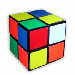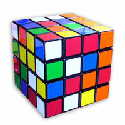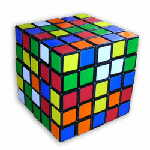### Cube Maths

#### Number of States (Arrangements)

File cubestates.pdf is an update of a document I wrote but did not publish in 1991. It examines the possible arrangements for cubes of any size n x n x n and includes numerical data for standard cubes (with unmarked centres) for the size range 2x2x2 to 25x25x25. Mathematical expressions for reachable states for the marked centres' option are also provided. Define m as the number of arrangements and n as the cube size.

It is widely accepted that for n = 3, m = 43,252,003,274,489,856,000 or m = 4.325 x 1019 approximately. If we define y = log10m, then for the 3x3x3 cube:

y = 19 + log10(4.3252003274490) = 19.6360062271976

The most significant result presented in the cubestates.pdf document is that y can be related to n by a continuous quadratic function subject to the restriction that n must be an integer greater than 1 when referring to possible arrangements for cubes:

y = An2 + Bn + C

where A, B and C are constants. Constants A and B are the same for n even and for n odd but the value of C is different. The following is a summary of some of the main values derived.

 Parameter Value A 3.87785955497335 B -3.61508538481188 CEVEN -1.71610938550614 CODD -4.41947361312695 Value of y for 16x16x16 cube 933.1745705306815

In graphical terms, when y is plotted, two parabolas of exactly the same shape are involved, with "even" cube values lying on one and "odd" cube values lying on the other. Use of the log function y provides the only practical means of plotting numbers that vary over such a huge range as that for the extended Rubik's cube. The slope of the y-n parabolic curves increase linearly with n. In practice, the variation caused by the difference in the value of C for n odd and n even is very small, so the two parabolas can be regarded as being nearly coincident.

With the logarithmic presentation, the number of cube states can be expressed using just four non-integer numbers (A, B and the two C values). Furthermore, the number of cube states form a restricted set of values for a more general continuous parabolic function for which n can have non-integer and negative values.

CDIFF (equal to CEVEN - CODD) has the value 2.70336422762081 which yields a numeric factor of 505.08471690483. That constant factor reflects the increased complexity of even relative to odd size cubes, which arises in addition to the main cube size-dependent factor.

All the above figures assume that there are no distinguishing features, such as a portion of an image on each centre cube element (cubie) within any given orbit, that would dictate the exact positions or orientations that these cubies need to occupy in the solved cube state. Expressions are also derived for the number of possible states that apply for such cubes with distinguishable centre cubies and, as a corollary, the number of different arrangements of centre cubies that apply for the solved state of the standard unmarked cube. It is clear that if you start with a standard unmarked set cube, scramble it and then unscramble it, then you have a negligible chance that all the centre cubies will be in the same location and orientation as they were in for the original set state.

The results of the analysis have no bearing on the methodology for solving the cube. Complete details are provided in file cubestates.pdf. The Excel 2003 files cubecalc.xls and cubecalc_mark.xls used for number crunching and chart plotting for cubes without and with marked centres respectively are also available. These files are also compatible with later versions of Excel.

#### Rubik's Family Cube Rules

Another document cuberules.pdf presents and justifies Rubik's family cube rules which in most cases need to be expressed as functions of cube size. The attributes considered are mostly mathematical in nature. Particular emphasis is given to parity and the number of unreachable states for cubes of any size (the number of reachable states are considered in the cubestates.pdf document noted above).

#### Permutation Cycle Length

A fundamental property of Rubik's family cubes of any size is that any permutation (move) repeated a sufficient number of times will result in the cube state returning to that which applied before the first application of the permutation. The permutation cycle length is the minimum number of times the permutation needs to be applied for the new state to correspond with the initial state. The cycle length is also referred to as the order of the permutation. The overall permutation comprising the defined permutation repeated the cycle length times represents an Identity Permutation.

Cycle length is likely to be of relevance only to those with an interest in cube mathematics. My Java Unravel program has a runtime selectable option for determining the cycle length of any user-nominated permutation for cubes of any size accommodated by the program. Smart moves (algorithms) tend to have short cycle lengths.

#### Other Mathematics for Rubik's Family Cubes

The major mathematics area for the standard size 3x3x3 Rubik's cube makes use of Group Theory to quantify the process involved in a rearrangement of cubies. The cuberules.pdf document provides references in that regard.